Courses
Courses for Kids
Free study material
Offline Centres
More

# Important Questions for CBSE Class 8 Maths Chapter 11 - MensurationLast updated date: 29th Nov 2023
Total views: 564.6k
Views today: 14.64k

## CBSE Class 8 Maths Important Questions for Mensuration - Free PDF Download

Math is a tricky subject to study, and thus having proper notes can boost up the studying procedure and eventually your grades. Important Questions for Class 8 Maths Chapter 11 is a student lifesaver consisting of all the necessary questions that might come in the exam. Professionals at Vedantu have especially curated these Class 8 Maths Chapter 11 Important Questions, keeping in mind the question paper's easy and difficult level. Students can get a quick revision before the exam by going through these Mensuration Class 8 Important Questions.

Vedantu is a platform that provides free (CBSE) NCERT Solution and other study materials to the students. Download Class 8 Maths and Class 8 Science NCERT Solutions to help you to revise complete syllabus and score more marks in your examinations.

## Study Important Questions for Class 8 Mathematics Chapter 11 – Mensuration

Very Short Answer Question                                                                      1 Mark

1.  Find the perimeter of the given figure

(a) ${\text{11cm}}$

(b) ${\text{8}}{\text{.5cm}}$

(c) ${\text{13cm}}$

(d) ${\text{9}}{\text{.5cm}}$

Ans: Perimeter of the given figure = ${\text{3cm + 3cm + 2}}{\text{.5cm = 8}}{\text{.5cm}}$

Therefore, the correct option is (b)

2. Area of a trapezium = Half of the sum of the length of parallel sides × _____?

Ans: Perpendicular distance between them.

3. The area of a parallelogram whose base is ${\text{9cm}}$and altitude is ${\text{6cm}}$

(a) ${\text{45c}}{{\text{m}}^{\text{2}}}$

(b) ${\text{54c}}{{\text{m}}^2}$

(c) ${\text{48c}}{{\text{m}}^{\text{2}}}$

(d) ${\text{84c}}{{\text{m}}^2}$

Ans: Area of parallelogram = base × altitude

= ${\text{9}}\;{\text{cm } \times 6}\;{\text{cm = 54}}\;{\text{c}}{{\text{m}}^{\text{2}}}$

Therefore, correct option is (b)

4. The volume of a cube whose edge is ${\text{6a}}$ is

(a) ${\text{25}}{{\text{a}}^{\text{3}}}$

(b) ${\text{216}}{{\text{a}}^3}$

(c) ${\text{125}}{{\text{a}}^{\text{3}}}$

(d) None of these

Ans: Volume of cube = ${\text{6}}{{\text{a}}^{\text{3}}}\;{\text{ = }}\;{\text{216}}{{\text{a}}^{\text{3}}}$

Therefore, the correct option is (c)

5. The sum of the areas of all six faces of a cuboid is the _____ of the cuboid.

(a) Volume

(b) Surface area

(c) Area

(d) Curved surface area

Ans:  Sum of the areas of all six faces of cuboid is the surface area of cuboid which is given by  ${\text{2(l b + b h + h l)}}$

Therefore, the correct option is (b)

6. The area of a. Rhombus is $240\mathrm{~cm}^{2}$ and one of the diagonals is $16 \mathrm{~cm}$ Then other diagonal is

(a) ${\text{25cm}}$

(b) ${\text{30cm}}$

(c) ${\text{18cm}}$

(d) ${\text{35cm}}$

Ans: Area of Rhombus= ${\text{240c}}{{\text{m}}^{\text{2}}}\;{\text{ = }}\;\dfrac{{\text{1}}}{{\text{2}}}{\text{(}}{{\text{d}}_{\text{1}}}{ \times }{{\text{d}}_{\text{2}}}{\text{)}}$

= ${\text{2} \times 240}\;{\text{c}}{{\text{m}}^{\text{2}}}\;{\text{ = }}\;{\text{16}}\;{\text{cm} \times }{{\text{d}}_{\text{2}}}$

$\Rightarrow $${{\text{d}}_{\text{2}}}{\text{ = }}\dfrac{{{\text{2}}\;{ \times }\;{\text{240}}\;{\text{c}}{{\text{m}}^{\text{2}}}}}{{{\text{16}}\;{\text{cm}}}}{\text{ = 30}}\;{\text{cm}} Therefore, correct option is (b) 7. The volume of water tank is {\text{3}}\;{{\text{m}}^{\text{3}}}. Its capacity in litres is (a) {\text{30}} (b) {\text{300}} (c) {\text{3000}} (d) None of these Ans: {\text{1}}{{\text{m}}^{\text{3}}}{\text{ = 1000}}\;{\text{litres}} V = {\text{3}}{{\text{m}}^{\text{3}}}{\text{ = 3(1000) = 3000}}\;{\text{litres}} Therefore, correct option is (c) Short Answer Questions 2 Mark 8. Find the area of a square, the length of diagonal is 2 \sqrt{2} m Ans: Area of Square = \dfrac{{\text{1}}}{{\text{2}}}{{\text{d}}^{\text{2}}}\;\;\;\;\;\;\;\;\;\;\;\;\;(\therefore {\text{d = 2}}\sqrt {\text{2}} {\text{m}}) where d = diagonal length Area of Square = \dfrac{{\text{1}}}{{\text{2}}}{{\text{(2}}\sqrt {\text{2}} \;{\text{m)}}^{\text{2}}}{\text{ = }}\dfrac{{\text{1}}}{{\text{2}}}\;{\times }\;{\text{4}}\;{ \times }\;{\text{2 }}{{\text{m}}^{\text{2}}}{\text{ = 4}}\;{{\text{m}}^{\text{2}}} 9. If the parallel sides of a parallelogram are {\text{2cm}}apart and their sum is {\text{12cm}} then find its area. Ans: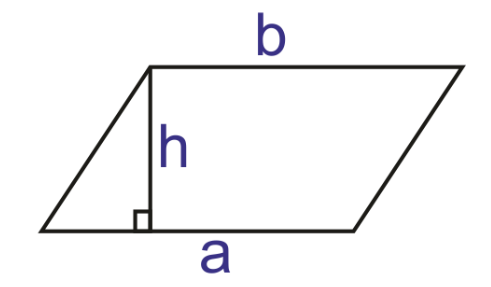Opposite sides of a parallelogram are equal {\text{a}}\;{\text{ + }}\;{\text{b}}\;{\text{ = }}\;{\text{12}} {\text{a}}\;{\text{ + }}\;{\text{a = 12}} {\text{2a = 12}} {\text{a = 6}} Area of parallelogram = {\text{a}}\;{ \times }\;{\text{h = 6}}\;{ \times }\;{\text{2 = 12}}\;{\text{c}}{{\text{m}}^{\text{2}}} 10. The length, breadth and height of a cuboid are {\text{20cm}}, {\text{15cm}} and {\text{10cm}} respectively. Find its total surface area. Ans: {\text{L = 20cm , B = 15cm , H = 10cm}} Surface area of cuboid = {\text{2(lb}}\;{\text{ + }}\;{\text{bh}}\;{\text{ + }}\;{\text{hl)}} = {{2(20 \times 15 + 15 \times 10 + 10 \times 20)c}}{{\text{m}}^{\text{2}}} = {\text{2(300}}\;{\text{ + }}\;{\text{150}}\;{\text{ + }}\;{\text{200)}}\;{\text{c}}{{\text{m}}^{\text{2}}} = {\text{2(650)}}\;{\text{c}}{{\text{m}}^{\text{2}}}{\text{ = 1300}}\;{\text{c}}{{\text{m}}^{\text{2}}} 11. Volume of Cube is {\text{8000c}}{{\text{m}}^{\text{3}}}. Find its surface area. Ans: V= {\text{8000}}\;{\text{c}}{{\text{m}}^{\text{3}}} {{\text{l}}^{\text{3}}}{\text{ = 8000}}\;{\text{c}}{{\text{m}}^{\text{3}}} \Rightarrow \;{\text{l = }}\sqrt[{\text{3}}]{{{\text{8000 c}}{{\text{m}}^{\text{3}}}{\text{ }}}}\; {\text{l}}\;{\text{ = }}\;{\text{20}}\;{\text{cm}} Surface Area of Cube = {\text{6}}{{\text{l}}^{\text{2}}} = {\text{6(20cm}}{{\text{)}}^{\text{2}}}{\text{ = 2400c}}{{\text{m}}^{\text{2}}} 12. Find the ratio of the areas of two circles whose radii is {\text{7cm}} and {\text{14cm}}. Ans: {{\text{r}}_{\text{1}}}{\text{ = 7cm}} \Rightarrow {{\text{A}}_{\text{1}}}{\text{ = }}\;\pi {{\text{(7)}}^{\text{2}}}{\text{ = 49}}\;\pi {{\text{r}}_2}{\text{ = 14cm}} \Rightarrow$${{\text{A}}_2}{\text{ = }}\pi \;{{\text{(14)}}^{\text{2}}}{\text{ = 196}}\;\pi$

${{\text{A}}_{\text{1}}}{\text{:}}{{\text{A}}_{\text{2}}}{\text{ = 49}}\pi \,{\text{:}}\;{\text{196}}\pi$

${{\text{A}}_{\text{1}}}{\text{:}}{{\text{A}}_{\text{2}}}{\text{ = 7:28}}$

13. Find the diameter of the circle whose circumference is ${\text{230m}}$.

Ans: Circumference = ${\text{230m}}$

${\text{2}}\pi {\text{r = 230}}\,{\text{m}}$

${\text{r = }}\dfrac{{{\text{230}}}}{{{\text{2}}\pi }}{\text{ = }}\dfrac{{{{230 \times 7}}}}{{{{2 \times 22}}}}{\text{ = 36}}{\text{.6}}\;{\text{m}}$

${\text{d}}\;{\text{ = }}\;{\text{2r}}\;{\text{ = }}\;{\text{2}}\;{{ \times }}\;{\text{36}}{\text{.6}}\;{\text{m}}\;{\text{ = }}\;{\text{73}}{\text{.18}}\;{\text{cm}}$

Question (14 – 18)                      3  Mark

14. Find the area of the figure if the upper portion is a semicircle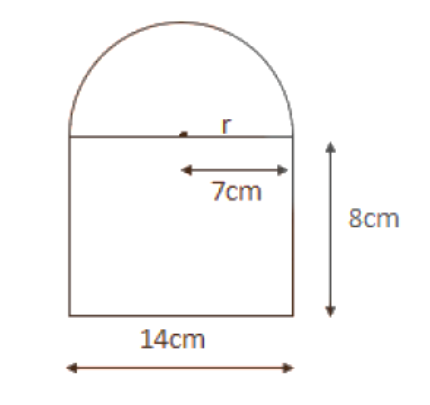Ans: Total area = Area of semicircle + Area of Rectangle

Area of semicircle = $\dfrac{{\text{1}}}{{\text{2}}}\pi \;{{\text{r}}^{\text{2}}}$

With ${\text{r = }}\dfrac{{\text{1}}}{{\text{2}}}{\text{(length}}\;{\text{of}}\;{\text{rectangle)}}\;{\text{ = }}\dfrac{{\text{1}}}{{\text{2}}}{{ \times 14 = 7}}$

Area of semicircle = $\dfrac{{\text{1}}}{{\text{2}}}{{ \times }}\dfrac{{{\text{22}}}}{{\text{7}}}{{ \times 7 \times 7 = 77}}\;{\text{c}}{{\text{m}}^{\text{2}}}$

Area of rectangle = length × breadth

= ${\text{14}}\;{{ \times }}\;{\text{8 = 112}}\;{\text{c}}{{\text{m}}^{\text{2}}}$

Total area = ${\text{(77}}\;{\text{ + }}\;{\text{112)}}\;{\text{c}}{{\text{m}}^{\text{2}}}{\text{ = 189}}\;{\text{c}}{{\text{m}}^{\text{2}}}$

15. A goat is tied to one corner of a square field of side ${\text{8m}}$by a rope ${\text{3m}}$ long. Find the area it can graze? Also find the area the goat cannot graze.

Ans: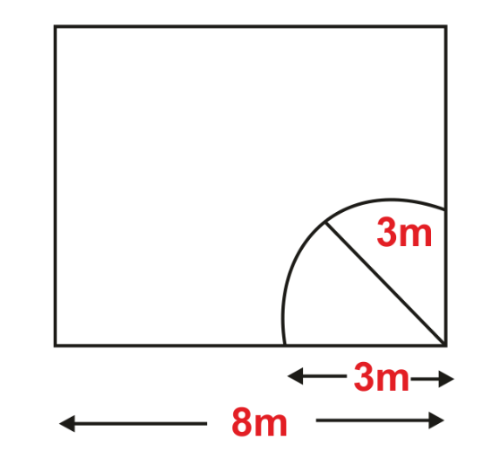Length of side of a square = ${\text{8}}\;{\text{m}}$

Area of square = ${{\text{(8}}\;{\text{m)}}^{\text{2}}}{\text{ = 64}}\;{{\text{m}}^{\text{2}}}$

Length of rope = ${\text{3m}}$ = ${\text{r}}$ (radius of circle)

As the goat is tied to a corner of square plot it can only graze $\dfrac{{\text{1}}}{{\text{4}}}{\text{th}}$ of circle of radius equal to length of rope inside the plot.

Area covered (or grazed) by goat = $\dfrac{{\pi \;{{\text{r}}^{\text{2}}}}}{{\text{4}}}$

= $\dfrac{{{\text{22}}}}{{\text{7}}}{ \times }\dfrac{{{{{\text{(3)}}}^{\text{2}}}}}{{\text{4}}}{\text{ = }}\dfrac{{{22 \times 9}}}{{{\text{28}}}}$

= ${\text{7}}{\text{.07}}\;{{\text{m}}^{\text{2}}}$

Area the goat cannot graze = Area of square – Area grazed by goat

= ${\text{64}}\;{\text{ - }}\;{\text{7}}{\text{.07 = 56}}{\text{.93}}\;{{\text{m}}^{\text{2}}}$

16. If ${\text{x}}$ units are added to the length of the radius of a circle, what is the number of units by which the circumference of the circle is increased?

Ans: Let the radius of the circle be ‘${\text{r}}$’ units

The circumference of the circle will be ${\text{2}}\pi \;{\text{r}}$ units

If the radius is increased by ‘${\text{x}}$’ units, the new radius will be ${\text{(r + x)}}$ units.

New circumference will be ${\text{2}}\pi \;{\text{(r}}\;{\text{ + }}\;{\text{x) = 2}}\pi \;{\text{r}}\;{\text{ + }}\;{\text{2}}\pi {\text{x}}$

Circumference increased by ${\text{2}}\pi \;{\text{x}}$ units.

17. Find the area of the shaded portion if diameter of circle is ${\text{16cm}}$ and ABCD is a square.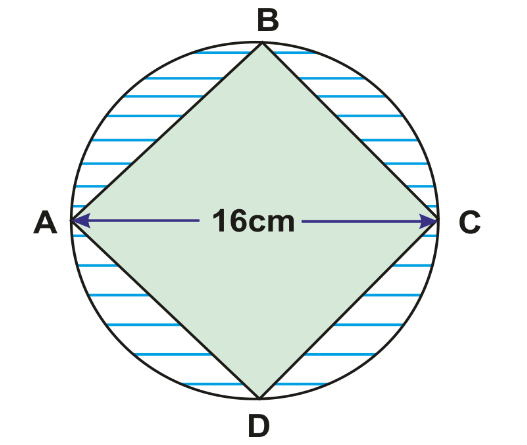Ans: Area of shaded portion = (Area of circle with radius =${\text{8cm}}$) – (Area of square with diagonal length = ${\text{16cm}}$)

= $\pi \;{{\text{r}}^{\text{2}}}{\text{ - }}\dfrac{{\text{1}}}{{\text{2}}}{{\text{d}}^{\text{2}}}$

= $\dfrac{{22}}{7} \times {(8)^2} - \dfrac{1}{2}{(16)^2}$

= $\dfrac{{22 \times 64}}{7} - 128$

= ${\text{201}}{\text{.1}}\;{\text{ - }}\;{\text{128}}\;{\text{ = }}\;{\text{73}}{\text{.1}}\;{\text{c}}{{\text{m}}^{\text{2}}}$

18. How many ${\text{c}}{{\text{m}}^{\text{3}}}$ of juice can be poured in a cuboidal can whose dimensions are $\text{15cm} \times \text{10cm} \times \text{25cm}$. How many cubical packs of ${\text{25c}}{{\text{m}}^{\text{3}}}$ volume can be made?

Ans: Volume of cuboid = Length $\times$ Breadth $\times$ Height

=  ${\text{15}}\;{\text{cm}}\;{ \times }\;{\text{10}}\;{\text{cm}}\;{\times}\;{\text{25}}\;{\text{cm}}$

Volume of juice in cuboidal can =  ${\text{3750}}\;{\text{c}}{{\text{m}}^{\text{3}}}$

Each volume of cubical packet =   ${\text{25}}\;{\text{c}}{{\text{m}}^{\text{3}}}$

Number of such cubical packets made from the volume of juice in cuboidal can

${\text{n = }}\dfrac{{{\text{volume}}\;{\text{of}}\;{\text{juice}}\;{\text{in}}\;{\text{cuboidal}}\;{\text{can}}}}{{{\text{each}}\;{\text{cubical}}\;{\text{pack}}\;{\text{volume}}}}$

${\text{n = }}\dfrac{{{\text{3750}}\;{\text{c}}{{\text{m}}^{\text{3}}}}}{{{\text{25}}\;{\text{c}}{{\text{m}}^{\text{3}}}}}$

${\text{n = 150}}$ packets

Question (19 – 25)                      5  Mark

19. A rectangular piece of paper${\text{66}}\;{\text{cm}}$long and${\text{10}}\;{\text{cm}}$broad is rolled along the length to form a cylinder. What is the radius of the base and calculate volume of cylinder?

Ans: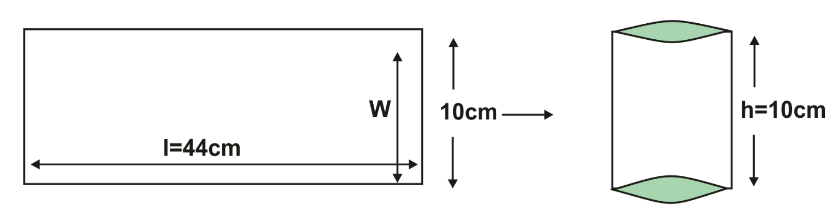When the rectangular piece is rolled in the form of a cylinder then the length became the circumference of the base of cylinder

C = ${\text{66}}$,

${\text{2}}\pi \;{\text{r = 66}}$

$\pi \;{\text{r = }}\dfrac{{{\text{66}}}}{{\text{2}}}{\text{ = 33}}$

${\text{r = }}\dfrac{{{\text{33}}\;{{ \times }}\;{\text{7}}}}{{{\text{22}}}}{\text{ = 10}}{\text{.5}}\;{\text{cm}}$

Volume of Cylinder with radius = ${\text{10}}{\text{.5cm}}$ ang height = ${\text{10cm}}$

${\text{V = }}\pi \;{{\text{r}}^{\text{2}}}{\text{h = }}\;\dfrac{{{\text{22}}}}{{\text{7}}}\;{{ \times }}\;{{\text{(10}}{\text{.5)}}^{\text{2}}}{{ \times }}\;{\text{10}}$

= ${\text{3465}}\;{\text{c}}{{\text{m}}^{\text{3}}}$

20. ABCD has area equal to ${\text{28}}$. BC is parallel to AD. BA is perpendicular to AD. If BC is ${\text{6}}$ and AD is ${\text{8}}$, then what is CD?

Ans: The shape of the given figure is a trapezium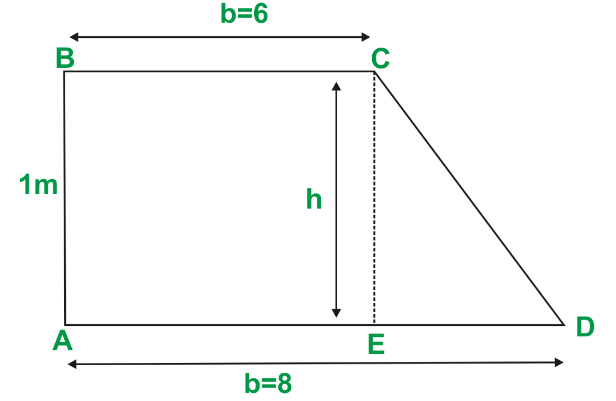Area of Trapezium = $\dfrac{1}{2}\text{(sum of parallel sides)} \times \text{height}$

Given area of ABCD = ${\text{28}}$, BC = ${\text{6}}$, AD = $8$, CD = ?

${\text{28 = A = }}\dfrac{{\text{1}}}{{\text{2}}}{\text{(6}}\;{\text{ + }}\;{\text{8)}}\;{\text{h}}$

${\text{h = }}\dfrac{{{\text{28}}\;{{ \times }}\;{\text{2}}}}{{{\text{14}}}}{\text{ = 4}}\;{\text{units}}$

To find CD: let DE perpendicular to AD (construction done)

In triangle CED,

ED = ${\text{8 - 6}}$

ED = ${\text{2}}$

${\text{C}}{{\text{D}}^{\text{2}}}{\text{ = C}}{{\text{E}}^{\text{2}}}{\text{ + E}}{{\text{D}}^{\text{2}}}$

${\text{C}}{{\text{D}}^{\text{2}}}{\text{ = }}\;{{\text{(4)}}^{\text{2}}}{\text{ + }}\;{{\text{(2)}}^{\text{2}}}{\text{ = 16}}\;{\text{ + }}\;{\text{4}}\;{\text{ = }}\;{\text{20}}$

${\text{CD = }}\sqrt {{\text{20}}} \;{\text{ = }}\;{\text{2}}\sqrt {\text{5}}$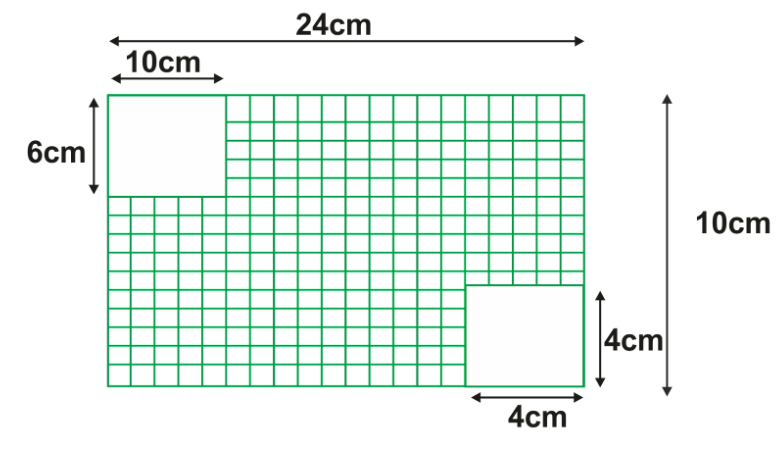Ans: From the figure,

Area of shaded portion = [Area of rectangle with ${\text{l = 24}}$, ${\text{b = 10}}$] – [Area of rectangle with ${\text{b = 6}}$, ${\text{l = 10}}$+ Area of square with side = ${\text{4}}$]

Area of big rectangle = ${\text{l}}\;{{ \times }}\;{\text{b}}$

= ${\text{24}}\;{{ \times }}\;{\text{10}}\;{\text{ = 240}}\;{\text{c}}{{\text{m}}^{\text{2}}}$

Area of small rectangle =  ${\text{l}}\;{{ \times }}\;{\text{b}}$

=   ${\text{6}}\;{{ \times }}\;{\text{10 }}\;{\text{ = }}\;{\text{60}}\;{\text{c}}{{\text{m}}^{\text{2}}}$

Area of squares = ${\text{4}}\;{{ \times }}\;{\text{4}}\;{\text{ = }}\;{\text{16}}\;{\text{c}}{{\text{m}}^{\text{2}}}$

Therefore, Area of shaded portion = ${\text{240}}\;{\text{ - }}\;{\text{(60}}\;{\text{ + }}\;{\text{16)}}$

= ${\text{240}}\;{\text{ - }}\;{\text{76 = 164}}\;{\text{c}}{{\text{m}}^{\text{2}}}$

22. A flooring tile has a shape of a parallelogram whose base is ${\text{28cm}}$ and the corresponding height is ${\text{20cm}}$. How many such tiles are required to cover a floor of area ${\text{2800}}{{\text{m}}^{\text{2}}}$.

Ans: Given, Base = ${\text{28cm}}$ , height = ${\text{20cm}}$

Area of floor =  ${\text{2800}}\;{{\text{m}}^{\text{2}}}$

= ${\text{2800}}\;\;{{ \times }}\;{\text{1}}{{\text{0}}^{\text{4}}}\;{\text{c}}{{\text{m}}^{\text{2}}}\;{\text{ = }}\;\;{\text{28}}\;{{ \times }}\;{\text{1}}{{\text{0}}^{\text{6}}}\;{\text{c}}{{\text{m}}^{\text{2}}}$

Area of each parallelogram tile = base × height

= ${\text{28}}\;{{ \times }}\;{\text{20 = 560}}\;{\text{c}}{{\text{m}}^{\text{2}}}$

Number of tiles required =  $\dfrac{{{\text{Area}}\;{\text{of}}\;{\text{floor}}}}{{{\text{Area}}\;{\text{of}}\;{\text{tiles}}}}{\text{ = }}\dfrac{{{\text{28} \times 1}{{\text{0}}^{\text{6}}}}}{{{\text{560}}}}$  = $\dfrac{{{\text{1}}{{\text{0}}^{\text{5}}}}}{{\text{2}}}{\text{ = }}\dfrac{{{\text{100000}}}}{{\text{2}}}{\text{ = 50000}}$

23. Rain water which falls on a flat rectangular surface of length ${\text{6m}}$ and breadth ${\text{4m}}$ is transferred into a cylindrical vessel of internal radius ${\text{20cm}}$. What will be the height of water in the cylindrical vessel if the rain fall is ${\text{1cm}}$ (Take $\pi \;{\text{ = }}\;{\text{3}}{\text{.14}}$)

Ans: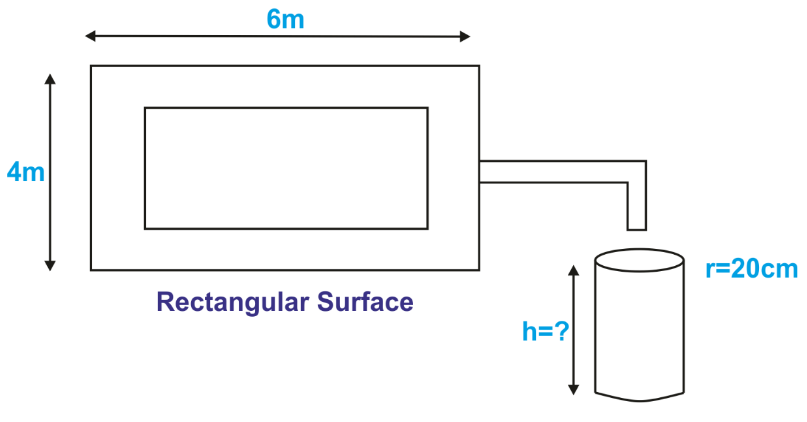Since the water in the rectangular surface is transferred to the cylindrical vessel.

Length of surface = ${\text{6}}\;{\text{m = 600}}\;{\text{cm}}$

Breadth of surface = ${\text{4}}\;{\text{m = 400}}\;{\text{cm}}$

Height of water level = ${\text{1}}\;{\text{cm}}$

Volume of water on the surface = ${\text{l}}\;{{ \times }}\;{\text{b}}\;{{ \times }}\;{\text{h}}$

\begin{align} & =\text{600}\ \times \ \text{400}\ \times \ \text{1} \\ & =\text{240000}\ \text{c}{{\text{m}}^{\text{3}}} \\ \end{align}

Let ‘${\text{h}}$’ be the height of the cylindrical vessel, ${\text{r = 20}}\;{\text{cm}}$ (radius of cylindrical vessel)

Volume of cylindrical vessel = $\pi \;{{\text{r}}^{\text{2}}}{\text{h}}$

= $\;\pi {{\text{(20)}}^{\text{2}}}\;{{ \times }}\;{\text{h}}$

Volume of water on surface = Volume of water in cylindrical vessel

${\text{24000 = }}\pi {{\text{(20)}}^{\text{2}}}{{ \times h}}$

${\text{h = }}\dfrac{{{\text{24000}}}}{{\pi \;{{ \times }}\;{\text{20}}\;{{ \times }}\;{\text{20}}}}{\text{ = 191}}{\text{.08}}\;{\text{cm}}$

24. If each edge of a cube is doubled

(a) how many times will its surface area increases

(b) how many times will its volume increases

Ans: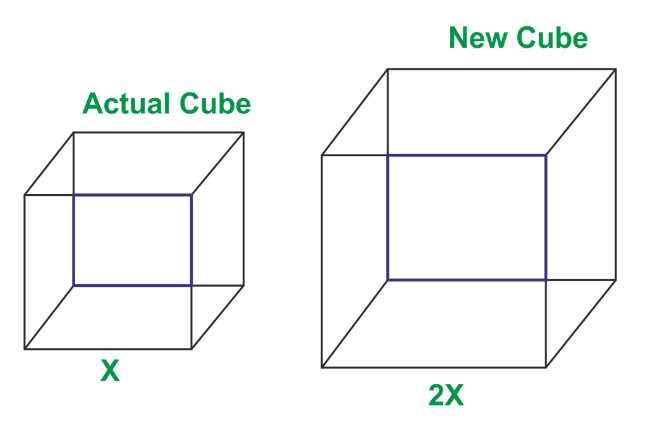For side of ‘x’ units, surface area ${{\text{s}}_{\text{1}}}\;{\text{ = }}\;{\text{6}}{{\text{x}}^{\text{2}}}$

When side of cube is doubled (${\text{2x}}$ units)

Surface area ${{\text{s}}_{\text{2}}}{\text{ = }}\;{\text{6(2x}}{{\text{)}}^{\text{2}}}$

${{\text{s}}_{\text{2}}}{\text{ = }}\;{\text{6}}\;{{ \times }}\;{\text{4(x}}{{\text{)}}^{\text{2}}}\;{\text{ = }}\;\;{\text{4(6}}{{\text{x}}^{\text{2}}}{\text{)}}\;{\text{ = }}\;{\text{4}}{{\text{s}}_{\text{1}}}$

Surface area increases by ${\text{4}}$ times.

Volume for edge of ‘${\text{x}}$’ units ${{\text{v}}_{\text{1}}}\;{\text{ = }}\;{{\text{x}}^{\text{3}}}$

Volume of cube when edge is doubled ${\text{(2x)}}$, ${{\text{v}}_{\text{2}}}\;{\text{ = }}\;{{\text{(2x)}}^{\text{3}}}$

${{\text{v}}_{\text{2}}}{\text{ = }}\;\;{\text{8(x}}{{\text{)}}^{\text{3}}}\;{\text{ = }}\;{\text{8}}{{\text{v}}_{\text{1}}}$

Therefore, volume increases by 8 times.

25. A box with measures ${\text{80cm} \times \text{48cm} \times \text{24cm}}$ is to be covered with a tarpaulin cloth how many metres of tarpaulin cloth of width ${\text{96cm}}$ is required to cover ${\text{50}}$ such boxes?

Ans: The box with ${\text{l}}\;{\text{ = }}\;{\text{80}}\;{\text{cm,}}\;{\text{b}}\;{\text{ = }}\;{\text{48}}\;{\text{cm,}}\;{\text{h}}\;{\text{ = }}\;{\text{24}}\;{\text{cm}}$

Total surface area = ${\text{2(lb}}\;{\text{ + }}\;{\text{bh}}\;{\text{ + }}\;{\text{hl)}}$

= ${\text{2[(80}}\; \times \;48)\;{\text{ + }}\;{\text{(48}}\; \times \;{\text{24)}}\;{\text{ + }}\;{\text{(80}}\; \times \;{\text{24)]}}$

= ${\text{2[3840}}\;{\text{ + }}\;{\text{1152}}\;{\text{ + }}\;{\text{1920]}}$

= ${\text{2 = 13824}}\;{\text{c}}{{\text{m}}^{\text{2}}}$

Length of cloth required = ${\text{(}}\dfrac{{{\text{Area}}\;{\text{of}}\;{\text{box}}}}{{{\text{breadth}}}}{\text{)}}\;{{ \times }}\;{\text{50}}$

= ${\text{(}}\dfrac{{13824}}{{96}}{\text{)}}\;{{ \times }}\;{\text{50 = 144}}\; \times \;{\text{50}}$

= ${\text{7200}}\;{\text{cm = 72}}\;{\text{m}}$

Mensuration is a relatively easy chapter that can be completely studied by the student in a matter of hours, provided that he/she is determined and diligently practised. Important Questions For Class 8 Maths Mensuration can help students to prepare for their exam in no time. Below are the topics listed within the chapter and the questions are based on these topics.

### Topics Included in Class 8 Maths Chapter 11

Following are the main topics in chapter mensuration as the division in the textbook. These topics help the students strengthen their base knowledge about the chapter to answer related questions.

Mensuration deals with studying kinds of geometrical shapes; for example, it's an area, length, volume, and perimeters. Below is a list of the table containing the name of shapes and the formula to calculate the questioned value.

 Shape Area Perimeter Curved or Lateral Surface Area Total Surface Area Volume Rectangle Length × Breadth 2(Length + Breadth) Triangle (1/2) × Base × Heightwhere, a, b and c are the three sides of the triangle) a + b + c Parallelogram Base × Height 2(sum of adjacent sides) Circle πr2 2πrWhere, r = radius of the circle Trapezium ½ ×(a+b)×h  or ½ ×h (sum of 2 parallel sides) General quadrilateral ½ ×d×(h1+h2) Rhombus ½ × d1×d2 Cylinder 2πrh 2πr(r+h) πr2h Cuboid 2h(l+b) 2(lb+bh+lh) lbh Cube 4l2 6l2 l3

### Benefits of Using Important Questions of Class 8 Maths for Mensuration

The Class 8 Maths Mensuration Important Questions PDF by Vedantu is curated by some of the best math professors to help the students understand the foundational concepts in math. For Chapter 11 Mensuration, the important questions contain all the essential topics explained understandably.

Math is a subject that is connected to humanity ever since the dawn of time. Scientists have previously discovered that the Indus Valley system used a type of numbering system for trade and commerce motives. In the contemporary world, math is equally important, if not more. We use it in our day to day lives, for example, buying groceries from the local supermarket and calculating the total bill.

### Why is NCERT Class 8 Mensuration Chapter is Vital?

Class 8 Mensuration chapter enhances the core of conceptual knowledge. It gives a full view of the prescribed syllabus, as the NCERT textbook doesn't contain an intricate description of the syllabus and the solutions. Every critical theorem in the chapter is fully solved, which makes the concept significantly simpler for students.

### Conclusion

The above-mentioned Class 8 Maths Chapter 11 Important Questions are reliable as these are prepared by trusted educational professionals at Vedantu. Solve these questions before the exam to sharpen your mind and feel prepared.

## FAQs on Important Questions for CBSE Class 8 Maths Chapter 11 - Mensuration

1. How can we get perfect scores on the NCERT Solutions for Class 8 Maths Chapter 11 - Mensuration in class tests?

You may get perfect scores on class tests and in board exams by following the Solutions for Class 8 Maths Chapter 11 - Mensuration offered on the Vedantu website. For quick and simple review during quizzes and exams, these solutions are really essential. For learners, this is the best study material right now.

2. What key ideas are discussed in Chapter 11 of the NCERT Solutions for Class 8 Math?

NCERT Solutions for Class 8 Chapter 11 Maths' primary subject areas are:

 Sr.No Topic 11.1 Introduction 11.2 Let us Recall 11.3 Area of Trapezium 11.4 Area of a General Quadrilateral 11.5 Area of a Polygon 11.6 Solid Shapes 11.7 Surface Area of Cube, Cuboid and Cylinder 11.8 Volume of Cube, Cuboid and Cylinder 11.9 Volume of Cube, Cuboid and Cylinder

3. What is a trapezium?

A convex quadrilateral having exactly one set of opposite sides that are parallel to one another is called a trapezium. The trapezium is a two-dimensional shape that resembles a table when it is drawn on paper. A quadrilateral is a polygon in Euclidean geometry that has four sides and four vertices. A trapezium therefore also has four vertices, four angles, and four sides.

4. What is the difference between Volume and capacity?

The total amount of space an object occupies in three dimensions is indicated by its volume. The term "capacity" describes something's ability to contain, absorb, or receive an object (such as a solid substance, gas, or liquid). Both solid and hollow objects have volume.. The capability only applies to hollow things.

eg- Suppose, for instance, that there is a water-filled tank. Thus, the volume of a tank refers to the area that the tank and its water occupy, but the capacity relates to the amount of water required to fill the tank.

5. What is a cylinder and what is the formula for the cylinder's curved surface area?

One of the most fundamental curvilinear geometric shapes, a cylinder, has historically been a three-dimensional solid. It is regarded as a prism with a circle as its base in basic geometry. In several contemporary fields of geometry and topology, a cylinder can alternatively be characterised as an infinitely curved surface.

A cylinder's curved surface area is equal to 2πrh square units, where r is the radius of the cylinder's circular ends and h is its height.